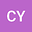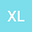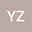A new complex structure-preserving method for QSVD
•••• CuiE Yu,
• Xin LIU,
• Yang Zhang
CuiE Yu
Macau University of Science and Technology

Corresponding Author:[email protected]

Author ProfileXin LIU
Macau University of Science and Technology
Author ProfileYang Zhang
Univ Manitoba
Author Profile## Abstract

In this paper, we propose a new structure-preserving algorithm for computing the singular value decomposition of a quaternion matrix $A$. We first define a quaternion-type matrix and prove that the multiplication of two quaternion-type matrices still be a quaternion-type matrix. Thus, utilizing this fact, we conduct a sequence of quaternion-type unitary transformations on a half of the elements of the complex adjoint matrix $\chi_A$ of $A$ instead of on the whole $\chi_A$. Then, we recover the resulting matrix with the help of the special structures. Compared with direct performing on the complex adjoint matrix, our algorithm needs only half of the computation and storage. This method also provides a novel proof for the existence of the singular value decomposition of a quaternion matrix. Moreover, numerical experiments are given to demonstrate the validity of our approach.